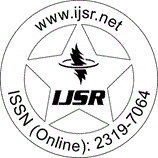International Journal of Science and Research (IJSR)
Call for Papers | Fully Refereed | Open Access | Double Blind Peer Reviewed

Research Paper | Mathematics | India | Volume 4 Issue 4, April 2015

# Numerical Solution of the First-Order Hyperbolic Partial Differential Equation with Point-Wise Advance

Chhatra Pal  | Vinit Chauhan 

Abstract: In this paper, we construct an explicit numerical scheme based on Lax-Friedrichs finite difference approximation to find the numerical solution of first-order hyperbolic partial differential equation with point-wise advance. The differential equation involving point-wise delay and advance models the distribution of the time intervals between successive neuronal firings. We construct higher order numerical approximation and discuss their consistency, stability and convergence. Analysis shows that numerical scheme is conditionally stable, consistent and convergent in discrete L-infinity norm. We also extend our method to the higher space dimensions. Some test examples are included to illustrate our approach. These examples verify the theoretical results and show the effect of point-wise advance on the solution.

Keywords: hyperbolic partial differential equation, transport equation, point-wise advance, finite difference method, Lax-Friedrichs scheme

Edition: Volume 4 Issue 4, April 2015,

Pages: 519 - 523